Finding Value of trignometric functions, given other functions

Chapter 3 Class 11 Trigonometric Functions
Concept wise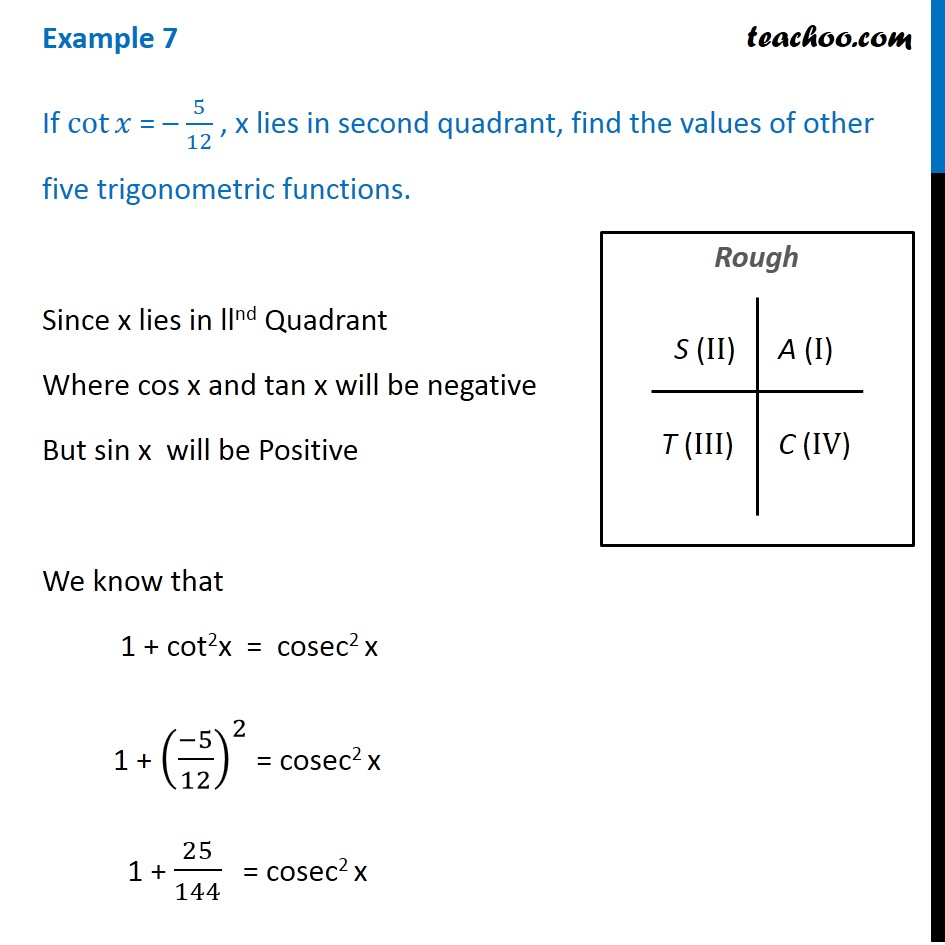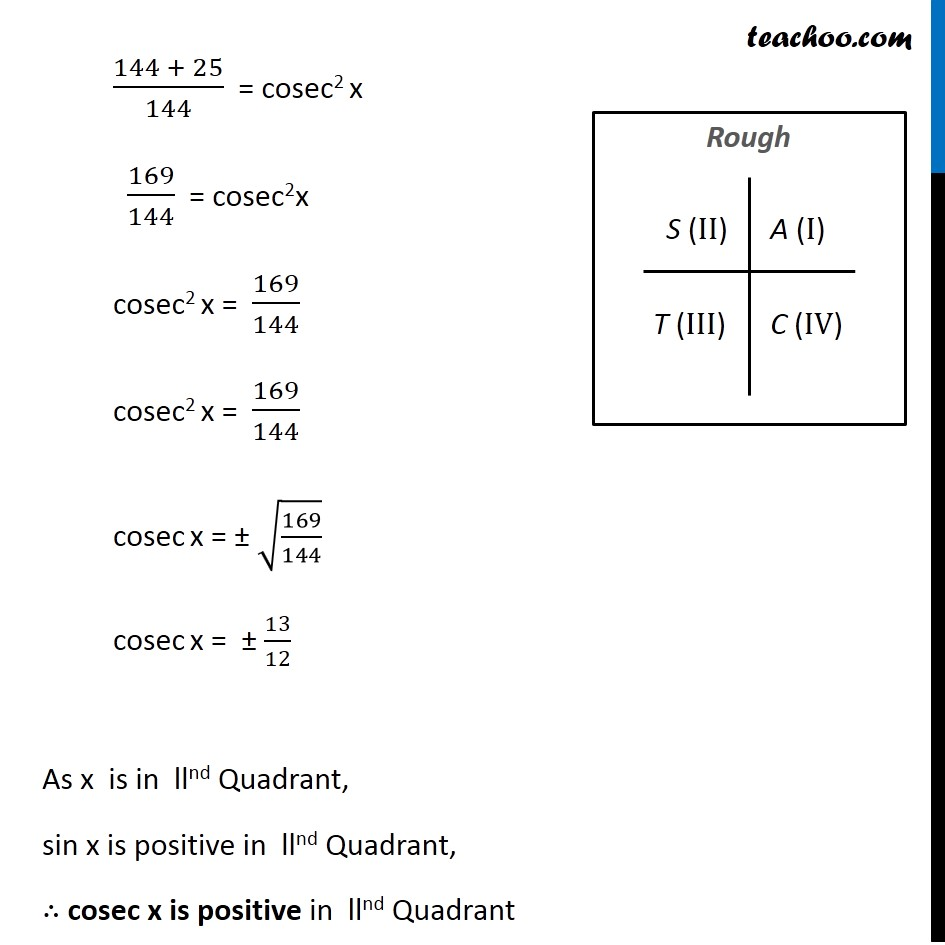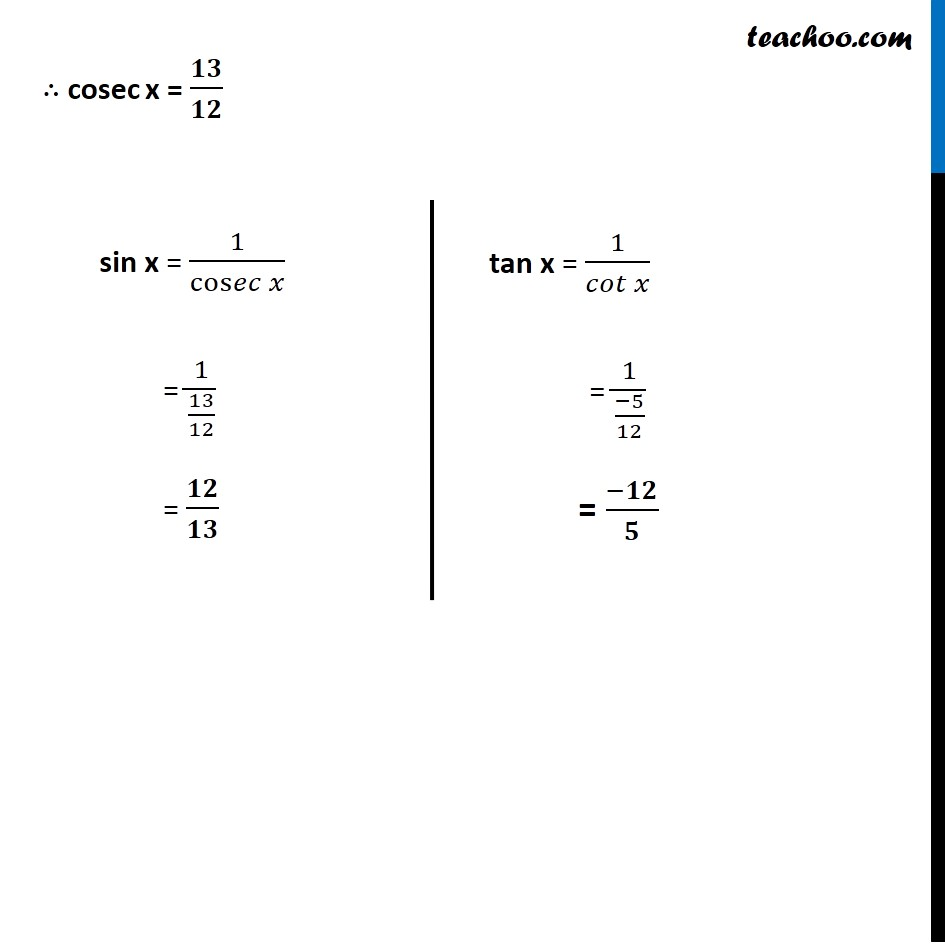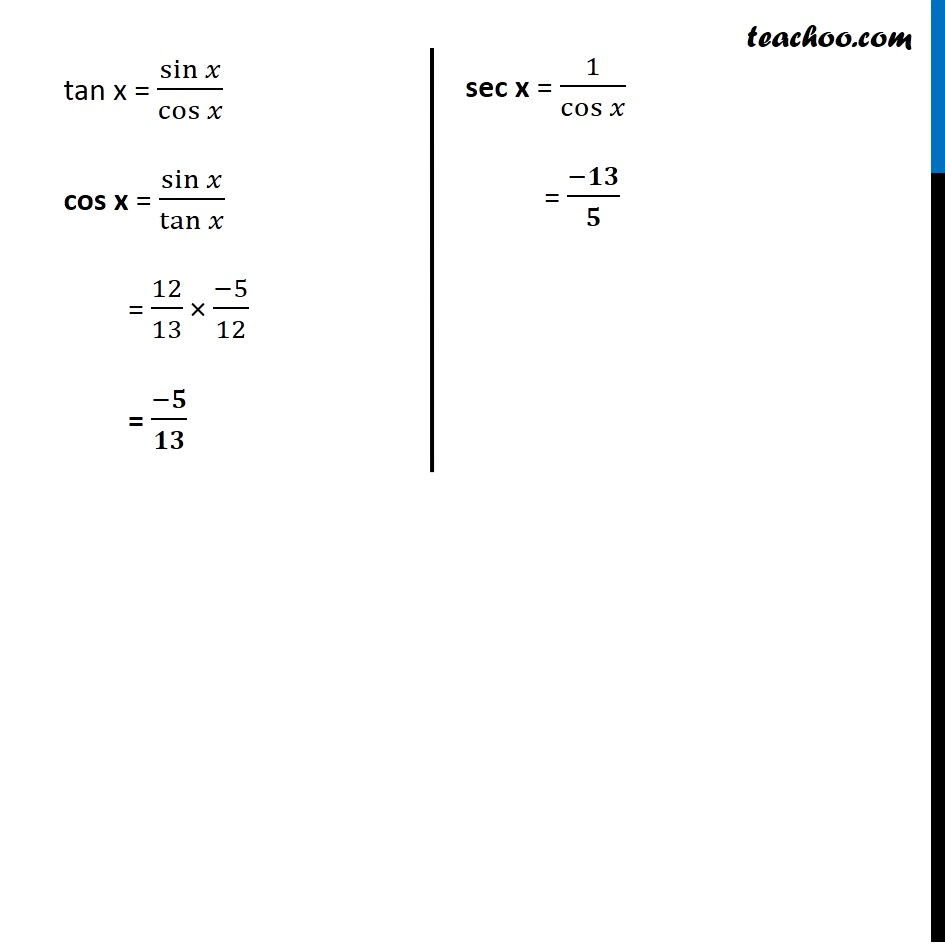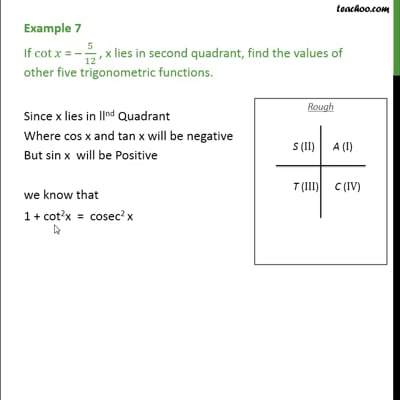This video is only available for Teachoo black users

Introducing your new favourite teacher - Teachoo Black, at only ₹83 per month

### Transcript

Example 7 If cot⁡𝑥 = – 5/12 , x lies in second quadrant, find the values of other five trigonometric functions. Since x lies in llnd Quadrant Where cos x and tan x will be negative But sin x will be Positive We know that 1 + cot2x = cosec2 x 1 + ((−5)/12)^2 = cosec2 x 1 + 25/144 = cosec2 x (144 + 25)/144 = cosec2 x 169/144 = cosec2x cosec2 x = 169/144 cosec2 x = 169/144 cosec x = ± √(169/144) cosec x = ± 13/12 As x is in llnd Quadrant, sin x is positive in llnd Quadrant, ∴ cosec x is positive in llnd Quadrant ∴ cosec x = 𝟏𝟑/𝟏𝟐 sin x = 1/cos𝑒𝑐⁡𝑥 = 1/(13/12) = 𝟏𝟐/𝟏𝟑 tan x = 1/(𝑐𝑜𝑡 𝑥) = 1/((−5)/12) = (−𝟏𝟐)/𝟓 tan x = sin⁡𝑥/cos⁡𝑥 cos x = sin⁡𝑥/tan⁡𝑥 = 12/13 × (−5)/12 = (−𝟓)/𝟏𝟑